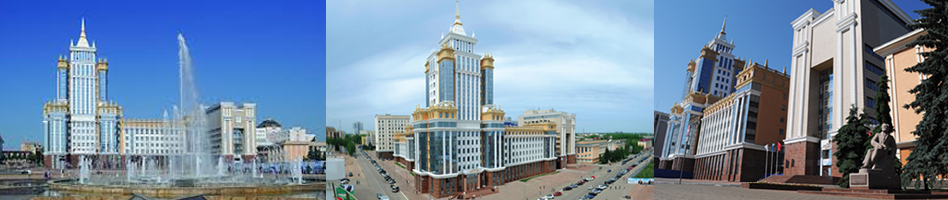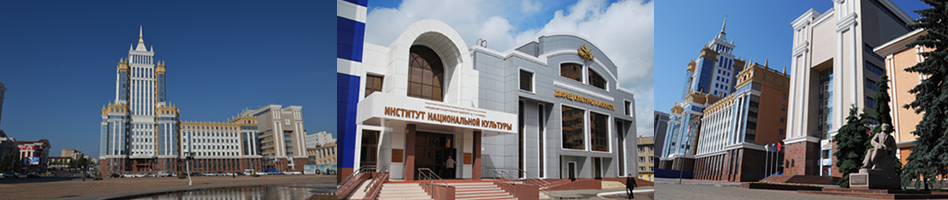To download article.

UDK 517.91

DOI: 10.15507/0236-2910.028.201803.344-351

Stability with Respect to a Part of Variables under Constant Perturbations of the Partial Equilibrium Position of Differential Equation Nonlinear Systems

Pavel P. Lipasov
Маster Degree Student of Chair of Fundamental Informatics and Information Technologies, National Research Mordovia State University (68/1 Bolshevistskaya St., Saransk 430005, Russia), ResearcherID: N-7266-2018, ORCID: https://orcid.org/0000-0001-9524-7353, This email address is being protected from spambots. You need JavaScript enabled to view it.

Professor of Chair of Applied Mathematics, Differential Equations and Theoretical Mechanics, National Research Mordovia State University (68/1 Bolshevistskaya St., Saransk 430005, Russia), D. Sc. (Physics and Mathemetics), ResearcherID: Q-3912-2018, ORCID: http://orcid.org/0000-0001-5230-3482, This email address is being protected from spambots. You need JavaScript enabled to view it.

Introduction. It is impossible to take into account all the forces acting in the process of mathematical modeling of dynamic processes. In order that mathematical models the most accurately describe the dynamic processes, they must include the terms that correspond the constant perturbations. These problems arise in applied tasks. In this paper we consider the case when the system allows for the partial equilibrium position. The aim of this work is to prove the stability theorem for the partial equilibrium position at constant perturbations, which are small at every instant.
Materials and Methods. The research objects are nonlinear systems of differential equations that allow for a partial equilibrium position. Using the second Lyapunov method, there are proved the stability theorems for the constant perturbations of the partial equilibrium position, which are small at every instant.
Results. Together with the introduction of stability for a part of the variables, it has become necessary to introduce stability for the part of phase variables under constant perturbations. The first stability theorem of the part of phase variables under constant perturbations was obtained by A. S. Oziraner. In this work, we prove a theorem of the stability of the constant perturbations of the partial equilibrium position, small at every instant. It should be noted that there is no stability theorems of constant perturbations for the partial equilibrium position. Thus, the theorem proved in this work is of a pioneer nature.
Conclusions. The theorem 3 proved in the work is the development of the mathematical theory of stability. The results of this work are applicable in the mechanics of controlled motion, nonlinear system.

Keywords: constantly acting disturbances, stability at constantly acting disturbances, partial equilibrium position, differential equation

For citation: Lipasov P. P., Schennikov V. N. Stability with Respect to a Part of Variables under Constant Perturbations of the Partial Equilibrium Position of Differential Equation Nonlinear Systems. Vestnik Mordovskogo universiteta = Mordovia University Bulletin. 2018; 28(3):344‒351. DOI: https://doi.org/10.15507/0236-2910.028.201803.344-351

Authors’ contribution: P. P. Lipasov – proof of theorem; V. N. Shchennikov – introduction of the research task, the definition of research methods.

All authors have read and approved the final version of the paper.

Received 18.04.2018; revised 07.06.2018; published online 20.09.2018

REFERENCES

1. Duboshin G. N. [On the question of the stability of the motion of relatively constant perturbations]. Tr. Gos. astronom. in-ta im. Shternberga = Proceedings of Shternberg State Astronomical Institute. 1940; 114:156–164 (In Russ.)

2. Malkin I. G. [On stability under constantly acting perturbations]. Prikladnaya matematika i mekhanika = Applied Mathematics and Mechanics. 1944; VIII(3):241–245 (In Russ.)

3. Krasovskiy N. N. [On the stability of motion as a whole with constantly acting perturbations]. Prikladnaya matematika i mekhanika = Applied Mathematics and Mechanics. 1954; 18:95–102 (In Russ.)

4. Tikhonov A. A. [On the stability of motion under constantly acting perturbations]. Vestnik Leningradskogo universiteta = Leningrad University Bulletin. 1965; 1:95–101 (In Russ.)

5. Gorshin S. I. [The second Lyapunov method and application to stability under constantly acting perturbations]. Vtoroy metod Lyapunova i yego primeneniye v energetike: tr. seminara-simpoziuma = The second Lyapunov method and its application in power engineering: Proceedings of symposium seminar. 1956; 1:5–34 (In Russ.)

6. Strauss A., Yorke A. J. Identifying perturbations which preserved asymptotic stability. Bull. Amer. Math. Soc. 1969; 22(2):513–518.

7. Corduneanu C. Sur la stabilite partielle. Rev. Roumaine math. Pures. Appl. 1964; 9(3):229–236.

8. Vorotnikov V. I. [Two classes of partial stability problems: to the unification of concepts and to unified solvability conditions]. Doklady RAN = Reports of RAS. 2002; 384(1):47–51 (In Russ.)

9. Vorotnikov V. I. [On stability and stability with respect to part of variable partial equilibrium positions of nonlinear dynamical systems]. Doklady RAN = Reports of RAS. 2003; 389(3):332–337.

10. Gabasov R. [On the stability of the solution of differential-operator equations under constantly acting perturbations]. Izvestiya vuzov. Matematika = Proceedings of Higher Schools. Mathematics. 1962; 30(5):29–38. (In Russ.)

11. Germaidze V. Ye., Krasovskiy N. N. [On stability under constantly acting perturbations]. Prikladnaya matematika i mekhanika = Applied Mathematics and Mechanics. 1957; 21(6):769–775 (In Russ.)

12. Gorshin S. I. [On some stability criteria for constantly acting perturbations]. Izvestiya vuzov. Matematika = Proceedings of Higher Schools. 1967; 11:17–20. (In Russ.)

13. Savchenko A. Ya. [On the stability of the motions of conservative mechanical systems under constantly acting perturbations]. Prikladnaya matematika i mekhanika = Applied Mathematics and Mechanics. 1974; 38(2):240–245 (In Russ.)